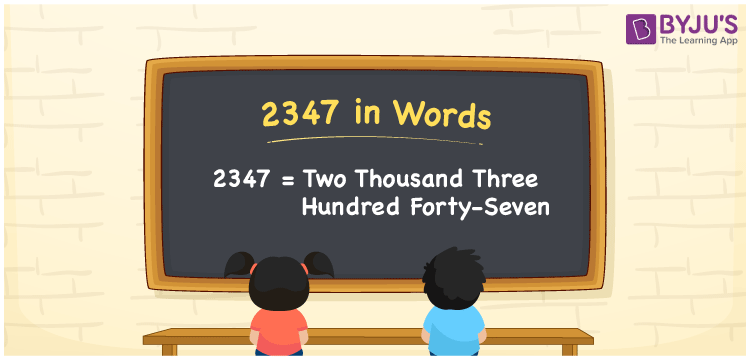# 2347 in Words

2347 in words is expressed as Two Thousand Three Hundred Forty-Seven. For example, the cost of a brass coffee filter is Rs. 2347, then you can write, “The cost of a brass coffee filter is Rupees Two Thousand Three Hundred Forty-Seven”. 2347 is a cardinal number since it represents a specific amount. Learn how to convert the number 2347 using a place value system with simple tricks in this article.

 2347 in Words Two Thousand Three Hundred Forty-Seven Two Thousand Three Hundred Forty-Seven in numerical form 2347

## 2347 in English Words

Generally, we write numbers in words using the letters of the English alphabet. Therefore, in English, we can read the number 2347 as Two Thousand Three Hundred Forty-Seven.## How to Write 2347 in Words?

The number 2347 is a four-digit number. So, we need a table of four columns to indicate its place value chart. The below table shows the place value chart for 2347.

 Thousands Hundreds Tens Ones 2 3 4 7

Hence, we can write the expanded form as:

2 x Thousand + 3 x Hundred + 4 x Ten + 7 x One

= 2 x 1000 + 3 x 100 + 4 x 10 + 7 x 1

= 2000 + 300 + 40 + 7

= 2347

= Two Thousand Three Hundred Forty-Seven

Therefore, 2347 in words is written as Two Thousand Three Hundred Forty-Seven

Interesting way of writing 2347 in words

2 = Two

23 = Twenty-Three

234 = Two Hundred and Thirty-Four

2347 = Two Thousand Three Hundred Forty-Seven

Thus, the word form of the number 2347 is Two Thousand Three Hundred Forty-Seven

2347 is a natural number that is one less than 2348 and one greater than 2346

• 2347 in words – Two Thousand Three Hundred Forty-Seven
• Is 2347 an odd number? – Yes
• Is 2347 an even number? – No
• Is 2347 a perfect square number? – No
• Is 2347 a perfect cube number? – No
• Is 2347 a prime number? – Yes
• Is 2347 a composite number? – No

## Frequently Asked Questions on 2347 in Words

Q1

### Write 2347 in words.

2347 in words is written as Two Thousand Three Hundred Forty-Seven.
Q2

### Simplify 1000 + 1347, and express in words.

Simplifying 1000 + 1347, we get 2347. Therefore, the number 2347 in words is Two Thousand Three Hundred Forty-Seven.
Q3

### Is 2347 a prime number?

Yes, the number 2347 is a prime number.